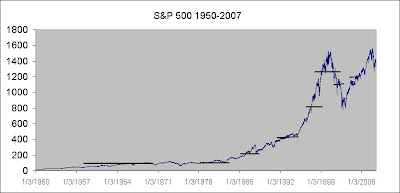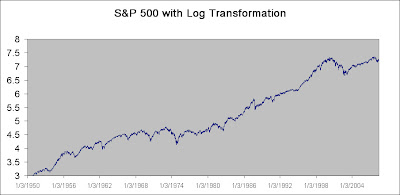## Thursday, February 4, 2010

### Practical Implementation of Neural Network based time series (stock) prediction -PART 4

Consider this an introduction to how we need to pre-process the data.
I mentioned earlier that a financial time series is typically a unit root or non-stationary signal, what this means is that if you sample statistical properties over time, they will obviously change.Fig 1. S&P 500 non-stationary signal

You can see that as we sample the average at various points it is constantly changing. Another property of a unit root time series is that it is continuously growing (or exploding). We need to somehow transform the time series back into a stationary signal, so that the Neural Net can process and learn it. Not only is it necessary for the Neural Net to see similar if not repeating data over and over, but any values beyond the internal squashing function will get saturated at the rails of the processing elements.

One of the things that you'll notice for many long term financial time series is that they grow exponentially, so a good candidate fit might be an exponential equation. However, since we will be using decomposition detrending, I prefer to use a line fit. In order to accomplish this, we can take the log of the data and later reverse the operation for post processing. Taking the log of exponential data also transforms the exponential regression to a linear one that we can use linear regression on and subtract the time series to get some stationarity .Fig 2. Log Transformed Time Series

Also, notice that we will be predicting the next day, so we can simply use linear regression parameters updated daily to predict the next day.

If we have a sufficient amount of data, we should see that the parameters settle to a stable limit, much as a coin toss converges to an asymptotic limit. If the parameters settle, we have some confidence that they will not change much from one sample prediction to the next.fig 3. Dynamic Slope Settling of Linear Prediction Parameters

Notice that the parameters have settled to a pretty stable value over the training period, implying that we don't expect them to change too wildly from the true value on the next predicted estimate.

After we subtract the line regression from the log transformed signal we get our detrended and stationary signal.fig 4. De-trended Log transformed signal

Notice it appears much more stationary than the original time series. However, because the Neural Network does not get to see a lot of repetitive high frequency information over the time window, I will detrend once more with a faster smoothed representation. First we will use a 100 period moving average as the new intermediate trend, then subtract a 25 period moving average to get the 2nd detrended series.Fig 5. Second de-trended series.

Notice that even this small sample shows a much better signal for the Neural Network to learn subtle patterns in the time series, and that stationarity property is very tame.FIg 6. Reconstructed prediction Out of Sample

The figure above shows an example of a stock series that has been decomposed and smoothed then recomposed with a 100 and 25 period moving average and the out of sample period. There is a very good correlation between predicted and actual smoothed estimates. Such a system might be utilized in a moving average crossover prediction to gain a 1 day advantage in estimating momentum. There are some very small discrepancies in predicted vs actual values, however, I believe it is due to one small problem I've had with Weka. The output of Weka only outputs 3 digits numerical precision. On the nabble forum they have mentioned a newer option in Subversion, but I haven't had a chance to play with it yet.

1.Hi,

May you give more details about who to do the first and second de-trend ?

I am trying to use NN to predict a time series, not financial, so I do 2 differencing of the serie and after I apply a log function. But the result is bad.

Serie Diff1 Diff2
3
5 5-3=2
6 6-5=1 1-2=-1
3 3-6=-3 -3-1=-4
4 4-3=1 1-(-3)=4

Maybe your process can give a better result.

Regards.

2.Kleyson,

I don't have a simple answer I'm afraid. But there are many different things to try; such as pre-processing data (scaling/normalizing), transforming, and or smoothing first.

IT